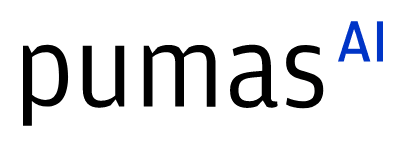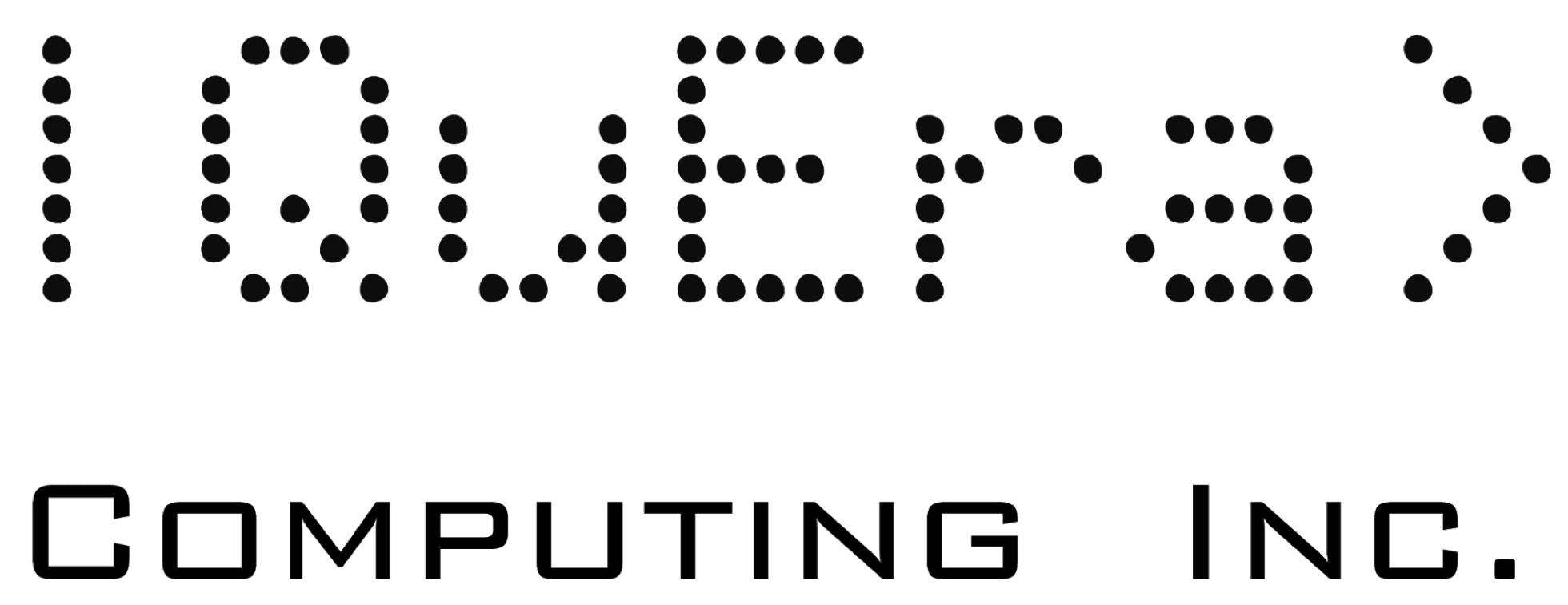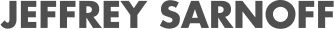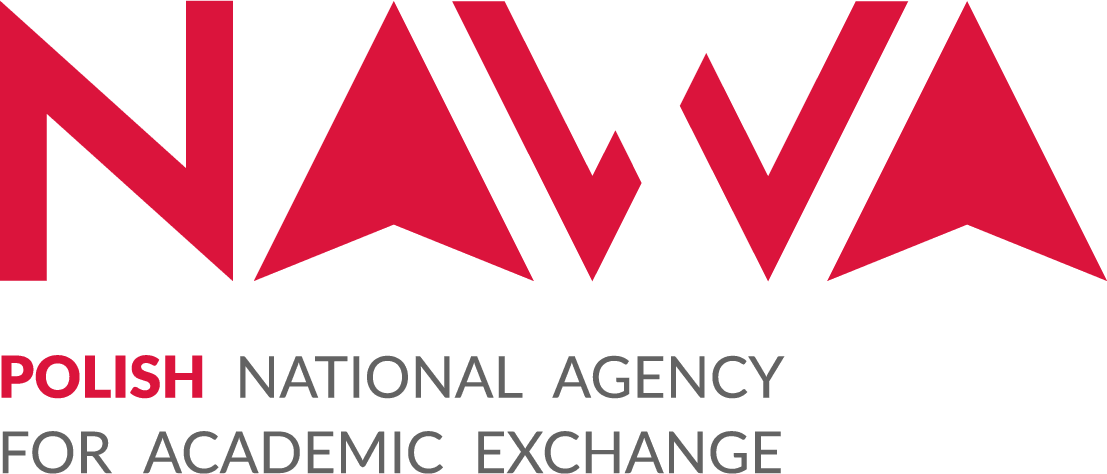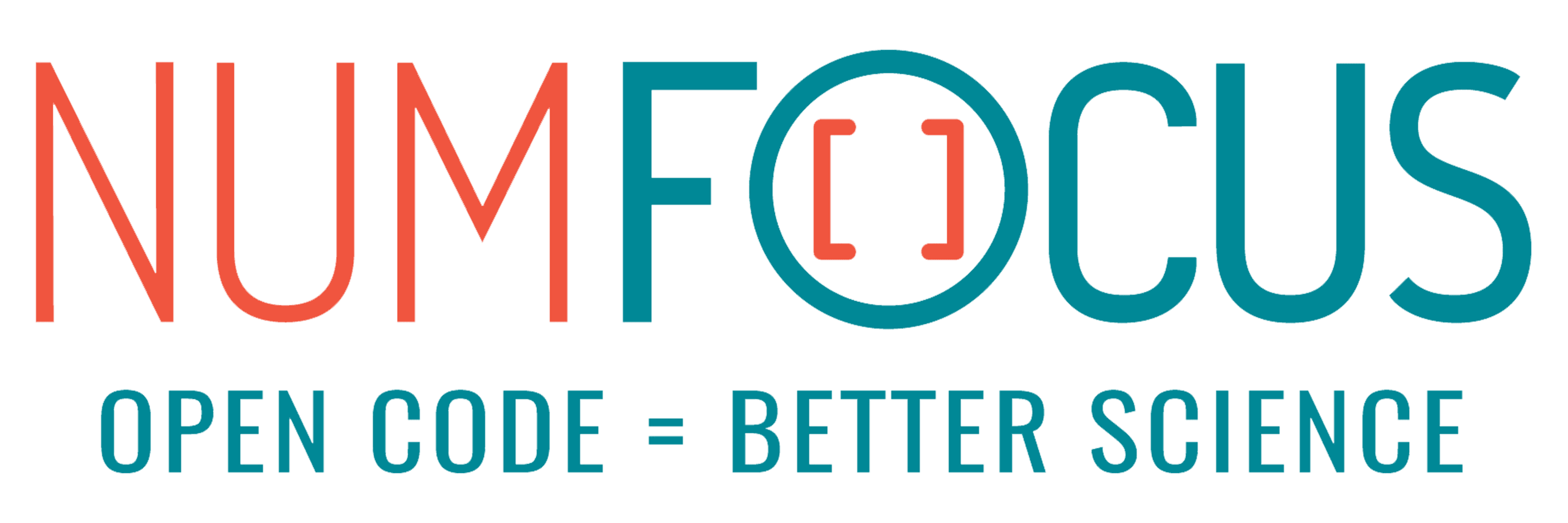# Fast Convex Optimization with GeNIOS.jl

07/28/2023, 2:50 PM3:00 PM UTC
32-155

#### Abstract:

We introduce GeNIOS.jl, a package for large-scale data-driven convex optimization. This package leverages randomized numerical linear algebra and inexact subproblem solves to dramatically speed up the alternating direction method of multipliers (ADMM). We showcase performance on a logistic regression problem and a constrained quadratic program. Finally, we show how this package can be extended to almost any convex optimization problem that appears in practice.

#### Description:

We introduce GeNIOS.jl, a Julia language alternating direction method of multipliers (ADMM) based convex optimization. We built this solver with an eye towards large-scale data-driven problems, such as those that that come up in robust optimization and machine learning. However, the solver can tackle any convex optimization problem that can be tractably solved with ADMM.

We first detail the algorithm. This solver builds on a line of work in randomized numerical linear algebra and inexact ADMM, i.e., ADMM in which the subproblems are only solved approximately. In GeNIOS.jl, we use a quadratic approximation to the smooth subproblem, an idea explored for the unconstrained case in . We then leverage the Nystrom Sketch to dramatically speedup the linear system solve  in this subproblem. When this subproblem comprises most of the computational effort, as is frequently the case in practice, our use of approximation and randomization yields significant speedups.

We will then introduce the package’s interface. We provide a `standard library’ which makes it easy to solve a number of problems, including logistic regression and quadratic programs. We will demonstrate how to define custom problems as well and show examples coming from different application areas. Finally, we will showcase the performance improvement of GeNIOS.jl compared to other methods.

This work builds on ideas presented at JuMP-dev last year, extending the class of problems tackled from quadratic programs to all convex optimization problems. We will conclude with more future directions and promising applications of these ideas and this solver.

 Zhao, S., Frangella, Z., & Udell, M. (2022). NysADMM: faster composite convex optimization via low-rank approximation. arXiv preprint arXiv:2202.11599.

 Frangella, Z., Tropp, J. A., & Udell, M. (2021). Randomized Nyström Preconditioning. arXiv preprint arXiv:2110.02820.